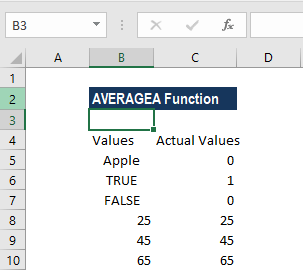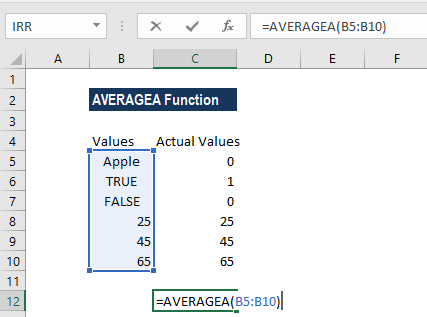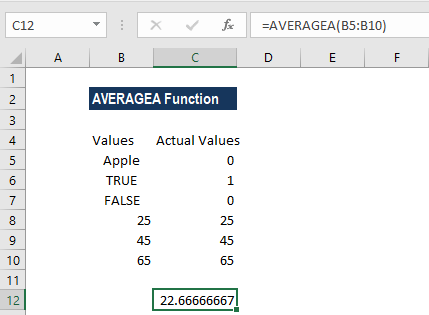# AVERAGEA Function

Calculates the average of a group of supplied values

## What is the AVERAGEA Function?

The AVERAGEA Function in Excel is a statistical function. The function calculates the average of a group of supplied values. It differs from the AVERAGE function, as it evaluates the logical values TRUE and FALSE, and numbers represented as text, whereas AVERAGE just skips these values during calculation. The AVERAGEA function was introduced in MS Excel 2007 and is not available in older versions.

### Formula

=AVERAGEA(value1, [value2], …)

Where:

1. Value1 is a required argument. However, subsequent values are optional.

Values that are supplied can be numbers, ranges, named ranges, or cell references that contain values. Up to 255 arguments can be supplied.

### How to use the AVERAGEA Function in Excel?

It is a built-in function which can be used as a worksheet function in Excel. To understand the uses of this function, let’s consider a few examples:

#### Example 1

With the arguments given below, let’s see how AVERAGEA function is used:As we can see in the above example:

• As shown in C5 – A cell with text in it would be considered as 0.
• As shown in B6 & B7 – A cell with FALSE in it is considered as 0 and TRUE is considered as 1.
• All cells with numbers would be taken as their actual numbers.
• The average value returned by the formula is 66666667. The formula returned is “(0+1+0+25+45+65)/6”.

#### Example 2

Let’s see a few more examples to understand the behavior of this function:As seen in the table above, we can see how each argument behaves when we apply the AVERAGEA function. The function can be supplied as a single number or cell, or as an array of numbers or cells.

The results are below:### Differences between AVERAGE and AVERAGEA Functions

ParticularsAVERAGE FunctionAVERAGEA Function
If we use logical values or text representations of numbers that are typed directly into the list of argumentsThey are counted as (TRUE=1, FALSE=0)They are counted as (TRUE=1, FALSE=0)
Text that cannot be interpreted as a number and are typed directly into the list of arguments#VALUE! error#VALUE! error
When we entered logical values within arrays or reference argumentsIgnored by this functionThey are counted as (TRUE=1, FALSE=0)
When we enter text that includes empty text "", text representations of numbers, or other text within arrays or reference argumentsIgnored by this functionThe function counts it as zero
Empty cellsIgnored by this functionIgnored by this function

### Things to remember in AVERAGEA Function:

1. AVERAGEA evaluates TRUE as 1 and FALSE as zero.
2. The arguments can be numbers, names, arrays, or references that contain numbers; text representations of numbers; or logical values, such as TRUE and FALSE, in a reference.
3. Arguments that contain TRUE are evaluated as 1 and arguments that contain FALSE are evaluated as 0 (zero).
4. If an argument is an array or reference, only values in that array or reference are used. Empty cells and text values in the array or reference are ignored.
5. The formula will return errors when the arguments are error values or text that cannot be translated into numbers.
6. If we do not wish to include logical values and text representations of numbers in a reference as part of the calculation, it would be better to use the AVERAGE function.

Thanks for reading CFI’s guide to important Excel functions! By taking the time to learn and master these functions, you’ll significantly speed up your financial analysis. To learn more, check out these additional CFI resources:

• Excel Functions for Finance